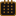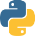# Articles | A collection of Articles, tutorials and thought processes | Olsen Portfolio

Saturday June 10th, 2023List Comprehensions

What is a list comprehension and why use it?

A list comprehension in Python is a way of creating a new list from an iterable object by performing an operation over each of its items! While it can seem quite odd and cryptic at first, it comes with benefits such as using less lines of code, making things more compact! Further more, it is faster than using say a conventional for-loop, because Python will allocate the list memory first, before adding the elements to it, (as opposed to having to resize on runtime). When fully understood, these lists become quite readable too!

We'll start off by using a simple example without using a list comprehension. Say we want to get all the squared numbers from 0 to 10. A traditional loop could look something like this:

Example 1a (using a for loop)``````square_numbers = [] # create an empty list
for i in range(11): # The last number within the range() method is not included
square_numbers.append(i * i)
print(square_numbers)

Output: [0, 1, 4, 9, 16, 25, 36, 49, 64, 81, 100]
``````

Example 1b (using a list comprehension instead)``````square_numbers = [i * i for i in range(11)]
print(square_numbers)

Output: [0, 1, 4, 9, 16, 25, 36, 49, 64, 81, 100]
``````

The basic formats of list comprehensions are as follows:

List Comprehension Syntaxes:

• new_list = [expression for item in iterable object]
• new_list = [expression for item in iterable object (if conditional)]
• new_list = [expression for item in iterable object (if conditional_1) and (if conditional_2)]
• new_list = [expression for item_1 in iterable object and item_2 in iterable object]

For clarity sake, it's important understand what each element of the syntaxes means:

• expression: This can be an item itself, a method, mathematical operation, a conditional statement or even another list comprehension! Ultimately, it returns a value. In example 1b, i * i is the expression.
• item: also known as a member, this is the object / value in the iterable object. In example 1b, the member value is i.
• iterable object: is an object that can return its elements one at a time. This can include a list, set, sequence or generator. In example 1b, the iterable object is range(11).
• conditional(s): optional - tests any valid expression. It be useful in filtering out unwanted values for example.

Let's examine a few more examples showcasing other various formats!

example 2 (using a conditional)``````even_numbers = [i for i in range(15) if i%2 == 0]
print(even_numbers)

Output: [0, 2, 4, 6, 8, 10, 12, 14]
``````

example 3a (using 'and' conditional)``````names = ["Steph", "Joey", "David", "Sarah", "Otis", "Julie", "Daniel", "Alexis", "Stan"]
name_fetch = [i for i in names if i.startswith("S") and i.endswith("h")]
print(name_fetch)

Output: ['Steph', 'Sarah']
``````

An alternative version of the code above can use two if conditionals back to back like so:

example 3b (using dual if conditionals)``````names = ["Steph", "Joey", "David", "Sarah", "Otis", "Julie", "Daniel", "Alexis", "Stan"]
name_fetch = [i for i in names if i.startswith("J") if i.endswith("y")]
print(name_fetch)

Ouput: ['Joey']
``````

example 4 (using dual if conditionals)``````numbers_list = [number for number in range(1, 11)]
squares = [n * n for n in numbers_list]
n_plus_square = [n + s for n, s in zip(numbers_list, squares)]
print(n_plus_square)

Output: [2, 6, 12, 20, 30, 42, 56, 72, 90, 110]
``````

Conclusion

Once understood, list comprehensions makes code more compact, yet easy to understand as well as perform faster than alternative ways! ∎